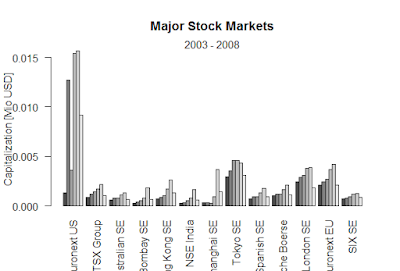## >How to apply univariate linear regression in data science with R.

We are going to predict quantitative response Y, is one predictor variable, x where why has a dinner relationship with x.
Y=b0+b1+e
b0=intercept
b1=slope
e=error term.
The least squares choose the model parameters that minimise the sum of square (RSS) of predict the value of x values versus the actual Y values.

data(anscombe)
>
> attach(anscombe)
>
> anscombe
x1 x2 x3 x4    y1   y2    y3    y4
1  10 10 10  8  8.04 9.14  7.46  6.58
2   8  8  8  8  6.95 8.14  6.77  5.76
3  13 13 13  8  7.58 8.74 12.74  7.71
4   9  9  9  8  8.81 8.77  7.11  8.84
5  11 11 11  8  8.33 9.26  7.81  8.47
6  14 14 14  8  9.96 8.10  8.84  7.04
7   6  6  6  8  7.24 6.13  6.08  5.25
8   4  4  4 19  4.26 3.10  5.39 12.50
9  12 12 12  8 10.84 9.13  8.15  5.56
10  7  7  7  8  4.82 7.26  6.42  7.91
11  5  5  5  8  5.68 4.74  5.73  6.89
> #correlation of x1 and y1
> cor(x1, y1)
 0.8164205
> cor(x2,y2)
 0.8162365
> cor(x3,y3)
 0.8162867
> cor(x4,y4)
 0.8165214
> cor(x2, y1)
 0.8164205
> #create a 2x2 grid for plotting
>FIG1

> par(mfrow=c(2,2))
> plot(x1, y1, main="Plot 1")
>
> plot(x2, y2, main="Plot 2")
>
> plot(x3, y3, main="Plot 3")
>
> plot(x4, y4, main="Plot 4")
> #Plot 1 appears to have a true linear relationship, Plot 2 is curvilinear, Plot
> 3 has a dangerous outlier, and Plot 4 is driven by the one outlier